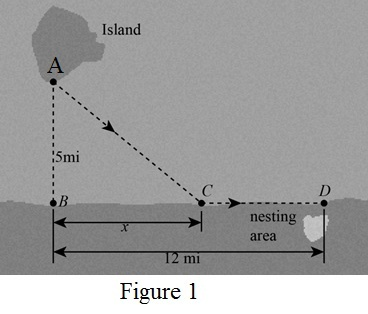# The total energy used by the bird is E ( x ) = 14 x 2 + 25 + 10 ( 12 − x ) .### Precalculus: Mathematics for Calcu...

6th Edition
Stewart + 5 others
Publisher: Cengage Learning
ISBN: 9780840068071### Precalculus: Mathematics for Calcu...

6th Edition
Stewart + 5 others
Publisher: Cengage Learning
ISBN: 9780840068071

#### Solutions

Chapter 2, Problem 31P

(a)

To determine

## To show: The total energy used by the bird is E(x)=14x2+25+10(12−x).

Expert Solution

### Explanation of Solution

Formula used:

energyused=energyper mile ×milesflown (1)

Calculation:

The energy of the bird requires 10kcal/mi to fly over the land and 14kcal/mi to fly over the water.

All the distances related to the bird are given in the figure below,Let x be distance between point B and C.

In ΔABC,

Use the Pythagoras theorem to find the length AC.

AC2=AB2+BC2[Hypothesis2=base2+perpendicular2]=(5)2+x2AC=25+x2

The length of the side BD is 12mi and the length of the side BC is x mi.

Then, the length of the side CD is,

CD=BDBC=12x

The bird flies from the point C to the point D on the land with the energy 10kcal/mi.

Energyforonemile=10kcal (2)

To find the energy for (12x)mile, multiply by 12x both side of the equation (2),

Energyfor(12x)mile=10(12x)kcal

The bird flies from the point A to the point C on the water with the energy 14kcal/mi.

Energyforonemile=14kcal (3)

To find the energy for 25+x2mile, multiply 25+x2 on both sides of the equation (3),

Energyfor25+x2mile=1425+x2kcal

Then the total energy is,

TotalEnergy=Energyfor(12x)mile+Energyfor25+x2mile (4)

Substitute 10(12x)kcal for Energyfor12xmile and 1425+x2kcal for Energyfor25+x2mile in the equation (4),

TotalEnergy=Energyfor(12x)mile+Energyfor25+x2mileE(x)=10(12x)kcal+1425+x2kcal=(10(12x)+1425+x2)kcal

Thus, the total energy is E(x)=14x2+25+10(12x).

(b)

To determine

### To find: The path that minimizes the energy expenditure.

Expert Solution

The bird flies till the point C on shoreline which is 5.013 miles from point B and then flies along the shoreline.

### Explanation of Solution

From the part (a), the total energy is E(x)=14x2+25+10(12x).

To find the minimum energy sketch the graph of E(x)=14x2+25+10(12x).

The function contains the variable x is the length of the side BC.

The local minimum value of the function is the least finite value where the value of the function at the any number is less than to the original function.

The condition for local minimum is,

f(a)f(x)

Using online graphing calculator, sketch the graph of the function E(x)=14x2+25+10(12x) as shown in the figure below.From the above figure, it can be observed that the least peak occurs at the point (5.103,168.99).

Then, the minimum energy expenditure is 168.99 at x=5.103.

Thus, the point C is 5.103 mi away from point B.

Therefore, the bird flies till the point C on shoreline which is 5.013 miles from point B and then flies along the shoreline.

### Have a homework question?

Subscribe to bartleby learn! Ask subject matter experts 30 homework questions each month. Plus, you’ll have access to millions of step-by-step textbook answers!